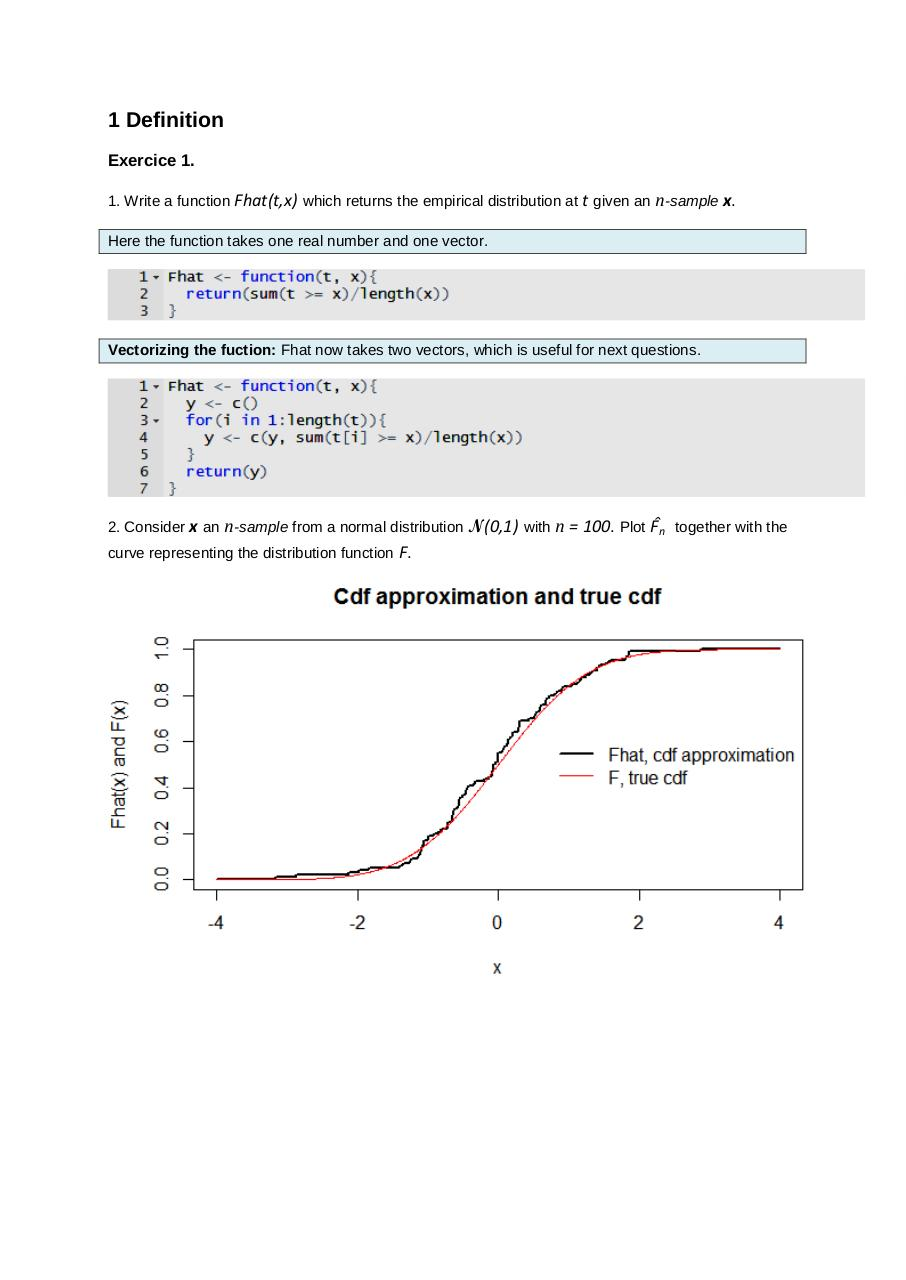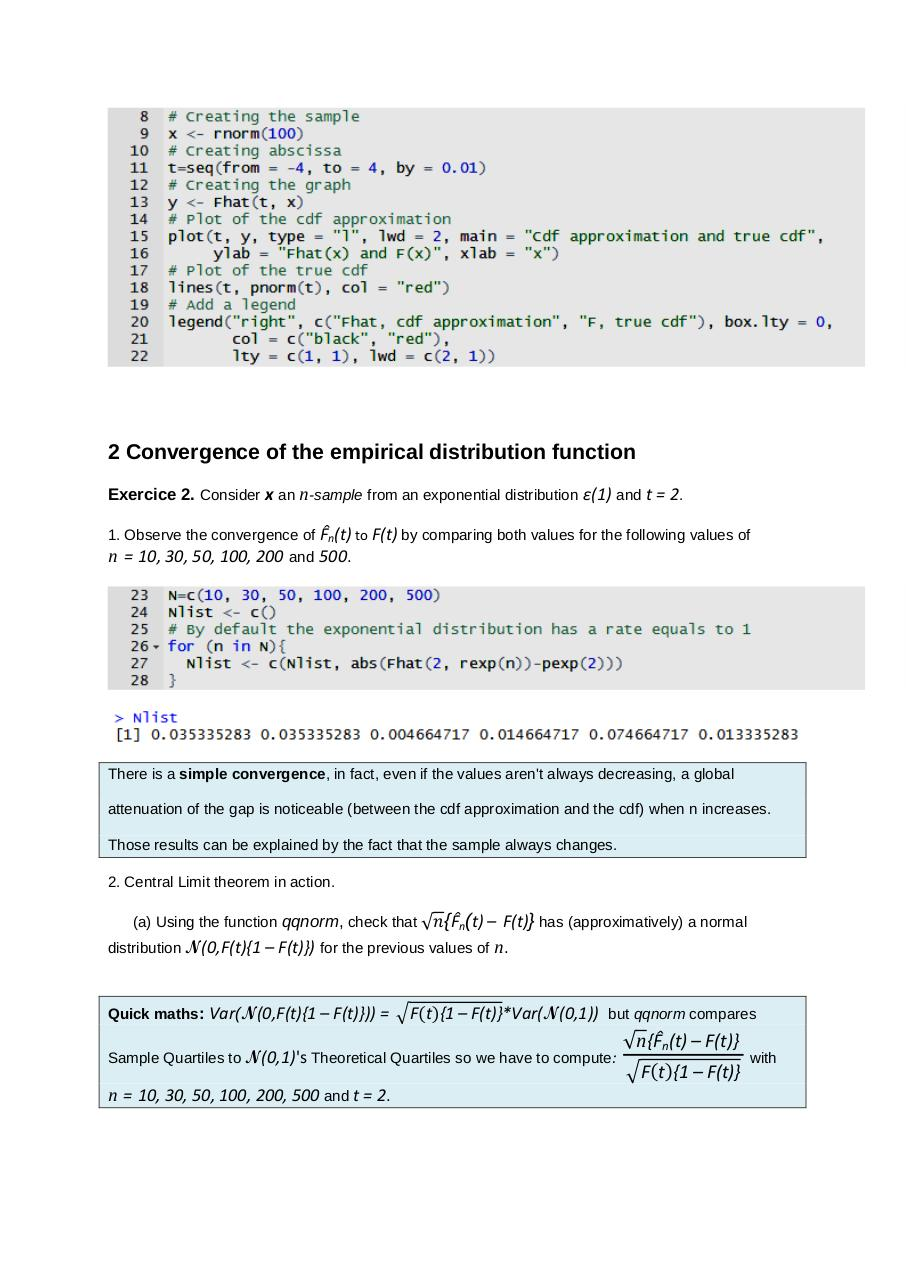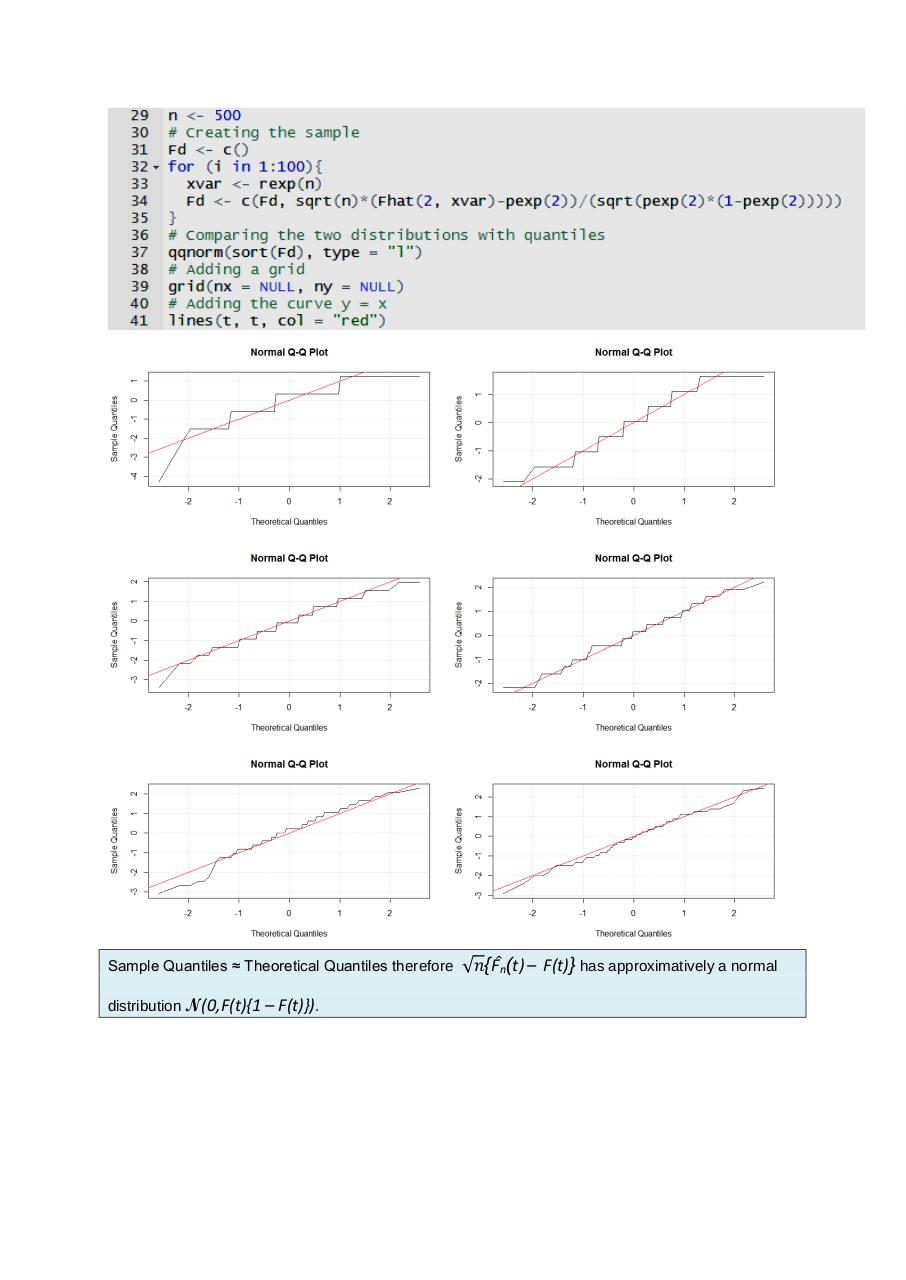# test .pdf

### File information

Original filename: test.pdf
Author: isabelle

This PDF 1.5 document has been generated by MicrosoftÂ® Word 2010, and has been sent on pdf-archive.com on 10/10/2017 at 17:27, from IP address 212.198.x.x. The current document download page has been viewed 661 times.
File size: 675 KB (3 pages).
Privacy: public file

test.pdf (PDF, 675 KB)

### Document preview

1 Definition
Exercice 1.
1. Write a function Fhat(t,x) which returns the empirical distribution at t given an n-sample x.
Here the function takes one real number and one vector.

Vectorizing the fuction: Fhat now takes two vectors, which is useful for next questions.

2. Consider x an n-sample from a normal distribution N(0,1) with
curve representing the distribution function F.

n = 100. Plot F̂n together with the

2 Convergence of the empirical distribution function
Exercice 2. Consider x an n-sample from an exponential distribution ε(1) and t = 2.
1. Observe the convergence of F̂n(t) to F(t) by comparing both values for the following values of
n = 10, 30, 50, 100, 200 and 500.

There is a simple convergence, in fact, even if the values aren't always decreasing, a global
attenuation of the gap is noticeable (between the cdf approximation and the cdf) when n increases.
Those results can be explained by the fact that the sample always changes.
2. Central Limit theorem in action.
(a) Using the function qqnorm, check that √𝑛{F̂n(t)

̶ F(t)} has (approximatively) a normal
distribution N(0,F(t){1 ̶ F(t)}) for the previous values of n.

Quick maths:

Var(N(0,F(t){1 ̶ F(t)})) = √F(t){1 ̶ F(t)}*Var(N(0,1)) but qqnorm compares

Sample Quartiles to N(0,1)'s Theoretical Quartiles so we have to compute:

n = 10, 30, 50, 100, 200, 500 and t = 2.

√n{F̂n(t) ̶ F(t)}
√F(t){1 ̶ F(t)}

with

Sample Quantiles ≈ Theoretical Quantiles therefore
distribution N(0,F(t){1

̶ F(t)}).

√𝑛{F̂n(t) ̶ F(t)} has approximatively a normal#### HTML Code

Copy the following HTML code to share your document on a Website or Blog

#### QR Code### Related keywords